# NCERT Solutions for Class 7 Maths Chapter 4 Simple Equations Ex 4.3

NCERT Solutions for Class 7 Maths Chapter 4 Simple Equations Ex 4.3

### NCERT Solutions for Class 7 Maths Chapter 4 Simple Equations Ex 4.3

NCERT Solutions for Class 7 Maths Chapter 4 Simple Equations Exercise 4.3
Ex 4.3 Class 7 Maths Question 1.
Solve the following equations: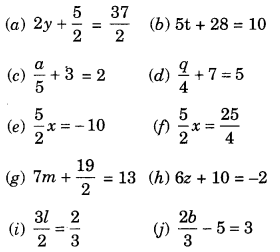Solution: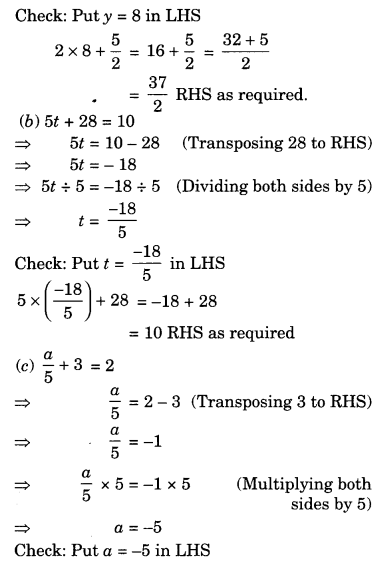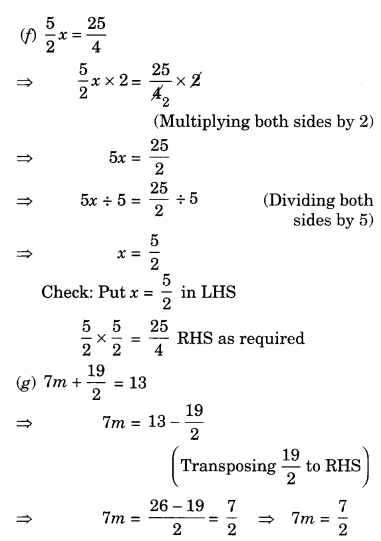Ex 4.3 Class 7 Maths Question 2.
Solve the following equations:
(а) 2(x + 4) = 12
(b) 3(n – 5) = 21
(c) 3(n – 5) = -21
(d) -4(2 + x) = 8
(e) 4(2 – x) = 8
Solution:
(a) 2(x + 4) = 12⇒ x = 6 – 4 (Transposing 4 to RHS)
⇒ x – 2
Check: Put x = 2 in LHS
2(2 + 4) = 2 × 6 = 12 RHS as required

(b) 3(n – 5) = 21⇒ n = 7 + 5 (Transposing 5 to RHS)
n = 12
Check: Put n = 12 in LHS
3(12 – 5) = 3 × 7 = 21 RHS as required

(c) 3(n – 5) = -21⇒ n = -7 + 5 (Transposing 5 to RHS)
⇒ n = -2
Check: Put n = -2 in LHS
3(-2 – 5) = 3 × -7
= -21 RHS as required

(d) -4(2 + x) = 8⇒ x = -2 – 2 (Transposing 2 to RHS)
⇒ x = —4
Check: Put x = -4 in LHS
-4(2 – 4) = -4 × -2 = 8 RHS as required

(e) 4(2-x) = 8∴ x = 0 (Multiplying both sides by -1)
Check: Put x = 0 in LHS
4(2 – 0) = 4 × 2 = 8 RHS as required

Ex 4.3 Class 7 Maths Question 3.
Solve the following equations:
(a) 4 = 5(p- 2)
(b) -4 = 5(p – 2)
(c) 16 = 4 + 3 (t + 2)
(d) 4 + 5(p – 1) = 34
(e) 0 = 16 + 4(m – 6)
Solution: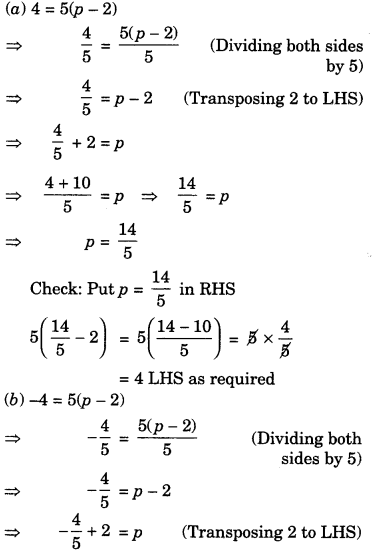(c) 16 = 4 + 3 (t + 2)(d) 4 + 5(p – 1) = 34(e) 0 = 16 + 4(m – 6)Ex 4.3 Class 7 Maths Question 4.
(a) Construct 3 equations starting with x = 2
(b) Construct 3 equations starting with x – -2.
Solution: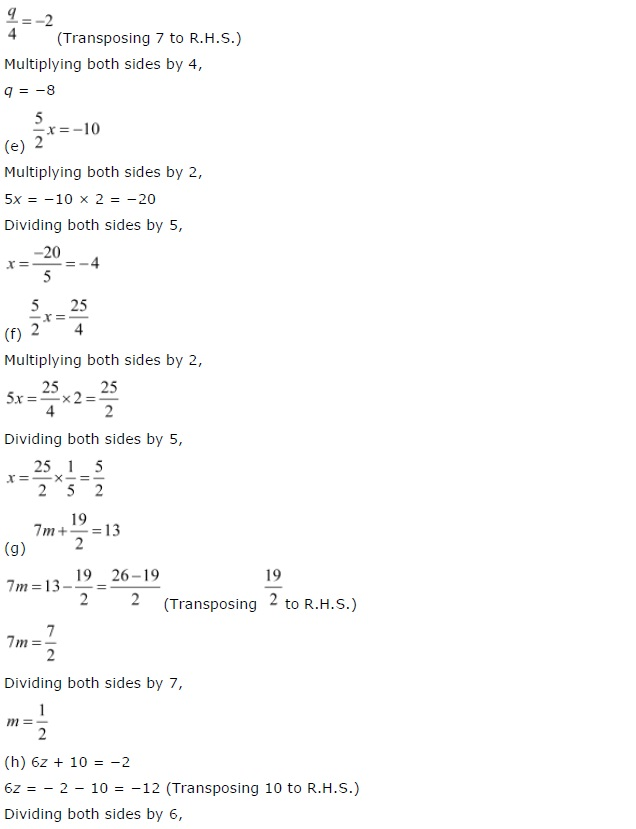## SabDekho

The Complete Educational Website